# 监督学习：从高维观察预测输出变量

scikit-learn 中所有监督的估计量 都有一个用来拟合模型的 fit(X, y) 方法，和根据给定的没有标签观察值 X 返回预测的带标签的 ypredict(X) 方法。

## 最近邻和维度惩罚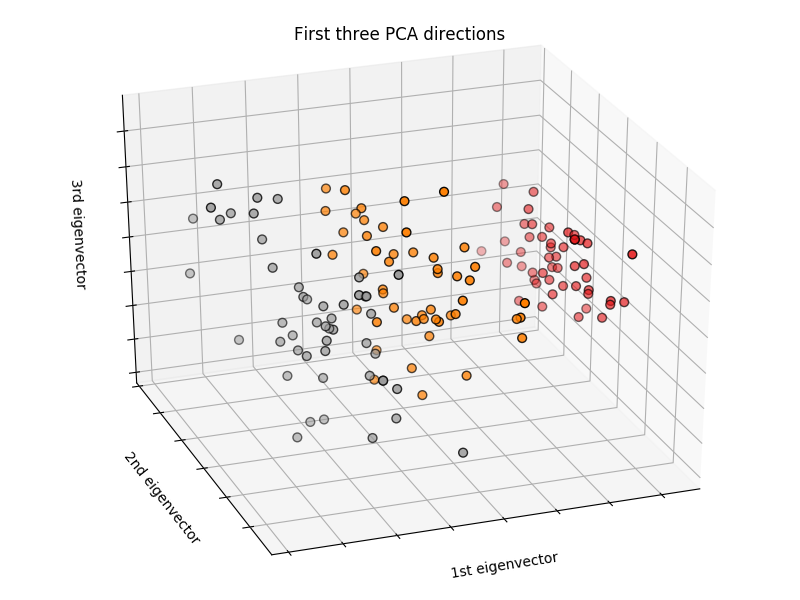py

import numpy as np from sklearn import datasets iris = datasets.load_iris() iris_X = iris.data iris_y = iris.target np.unique(iris_y) array([0, 1, 2])


### K近邻分类器

[最近邻](https://en.wikipedia.org/wiki/K-nearest_neighbor_algorithm): 也许是最简单的分类器：给定一个新的观察值 X_test，用最接近的特征向量在训练集(比如，用于训练估计器的数据)找到观察值。(请看 Scikit-learn 在线学习文档的 [最近邻章节](7#16-最近邻) 获取更多关于这种分类器的信息)

>**训练集和测试集**
>
>当用任意的学习算法进行实验时，最重要的就是不要在用于拟合估计器的数据上测试一个估计器的预期值，因为这不会评估在 **新数据** 上估计器的执行情况。这也是数据集经常被分为 _训练_ 和 _测试_ 数据的原因。

**KNN(k 最近邻)分类器例子**:

[![http://sklearn.apachecn.org/cn/0.19.0/_images/sphx_glr_plot_classification_001.png](img/86003b5287219bcbec1586985a110629.jpg)](https://scikit-learn.org/stable/auto_examples/neighbors/plot_classification.html)

py
>>> # 将鸢尾属植物数据集分解为训练集和测试集
>>> # 随机排列，用于使分解的数据随机分布
>>> np.random.seed(0)
>>> indices = np.random.permutation(len(iris_X))
>>> iris_X_train = iris_X[indices[:-10]]
>>> iris_y_train = iris_y[indices[:-10]]
>>> iris_X_test  = iris_X[indices[-10:]]
>>> iris_y_test  = iris_y[indices[-10:]]
>>> # 创建和拟合一个最近邻分类器
>>> from sklearn.neighbors import KNeighborsClassifier
>>> knn = KNeighborsClassifier()
>>> knn.fit(iris_X_train, iris_y_train)
KNeighborsClassifier(algorithm='auto', leaf_size=30, metric='minkowski',
metric_params=None, n_jobs=1, n_neighbors=5, p=2,
weights='uniform')
>>> knn.predict(iris_X_test)
array([1, 2, 1, 0, 0, 0, 2, 1, 2, 0])
>>> iris_y_test
array([1, 1, 1, 0, 0, 0, 2, 1, 2, 0])



## 线性模型：从回归到稀疏

py

diabetes = datasets.load_diabetes() diabetes_X_train = diabetes.data[:-20] diabetes_X_test = diabetes.data[-20:] diabetes_y_train = diabetes.target[:-20] diabetes_y_test = diabetes.target[-20:]

>
>任务是使用生理特征来预测疾病级别。

### 线性回归

[LinearRegression](../../modules/generated/sklearn.linear_model.LinearRegression.html#sklearn.linear_model.LinearRegression "sklearn.linear_model.LinearRegression")，最简单的拟合线性模型形式，是通过调整数据集的一系列参数令残差平方和尽可能小。

[![http://sklearn.apachecn.org/cn/0.19.0/_images/sphx_glr_plot_ols_001.png](img/7a9775b9051c948f74639f1856f6c585.jpg)](https://scikit-learn.org/stable/auto_examples/linear_model/plot_ols.html)

Linear models: ![y = X\beta + \epsilon](img/c5f2af9df9f65f0e399542ecf7f40554.jpg)

*   ![X](img/43c1fea57579e54f80c0535bc582626f.jpg): 数据
*   ![y](img/0775c03fc710a24df297dedcec515aaf.jpg): 目标变量
*   ![\beta](img/533e54759d696211ebe7819cc107d3bc.jpg): 回归系数
*   ![\epsilon](img/58ef9e1b5d2ee139dcb588a3879ca1a6.jpg): 观察噪声

py
>>> from sklearn import linear_model
>>> regr = linear_model.LinearRegression()
>>> regr.fit(diabetes_X_train, diabetes_y_train)
LinearRegression(copy_X=True, fit_intercept=True, n_jobs=1, normalize=False)
>>> print(regr.coef_)
[   0.30349955 -237.63931533  510.53060544  327.73698041 -814.13170937
492.81458798  102.84845219  184.60648906  743.51961675   76.09517222]

>>> # 均方误差
>>> np.mean((regr.predict(diabetes_X_test)-diabetes_y_test)**2)
2004.56760268...

>>> # 方差分数：1 是完美的预测
>>> # 0 意味着 X 和 y 之间没有线性关系。
>>> regr.score(diabetes_X_test, diabetes_y_test)
0.5850753022690...



### 收缩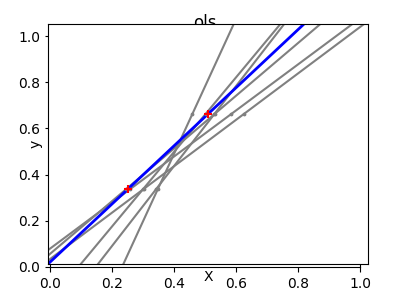>>> X = np.c_[ .5, 1].T
>>> y = [.5, 1]
>>> test = np.c_[ 0, 2].T
>>> regr = linear_model.LinearRegression()

>>> import matplotlib.pyplot as plt
>>> plt.figure()

>>> np.random.seed(0)
>>> for _ in range(6):
...    this_X = .1*np.random.normal(size=(2, 1)) + X
...    regr.fit(this_X, y)
...    plt.plot(test, regr.predict(test))
...    plt.scatter(this_X, y, s=3)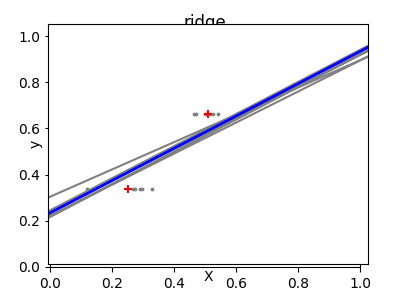>>> regr = linear_model.Ridge(alpha=.1)

>>> plt.figure()

>>> np.random.seed(0)
>>> for _ in range(6):
...    this_X = .1*np.random.normal(size=(2, 1)) + X
...    regr.fit(this_X, y)
...    plt.plot(test, regr.predict(test))
...    plt.scatter(this_X, y, s=3)



>>> alphas = np.logspace(-4, -1, 6)
>>> from __future__ import print_function
>>> print([regr.set_params(alpha=alpha
...             ).fit(diabetes_X_train, diabetes_y_train,
...             ).score(diabetes_X_test, diabetes_y_test) for alpha in alphas])
[0.5851110683883..., 0.5852073015444..., 0.5854677540698..., 0.5855512036503..., 0.5830717085554..., 0.57058999437...]



### 稀疏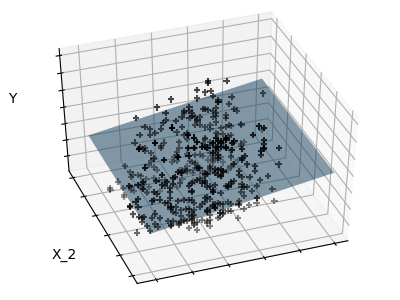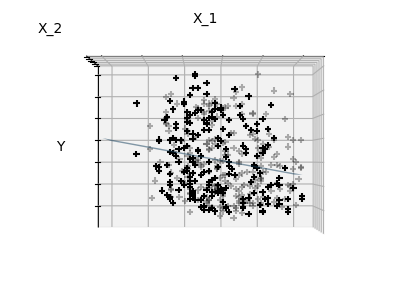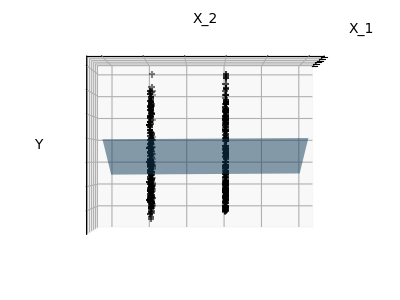>>> regr = linear_model.Lasso()
>>> scores = [regr.set_params(alpha=alpha
...             ).fit(diabetes_X_train, diabetes_y_train
...             ).score(diabetes_X_test, diabetes_y_test)
...        for alpha in alphas]
>>> best_alpha = alphas[scores.index(max(scores))]
>>> regr.alpha = best_alpha
>>> regr.fit(diabetes_X_train, diabetes_y_train)
Lasso(alpha=0.025118864315095794, copy_X=True, fit_intercept=True,
max_iter=1000, normalize=False, positive=False, precompute=False,
random_state=None, selection='cyclic', tol=0.0001, warm_start=False)
>>> print(regr.coef_)
[   0.         -212.43764548  517.19478111  313.77959962 -160.8303982    -0.
-187.19554705   69.38229038  508.66011217   71.84239008]



### 分类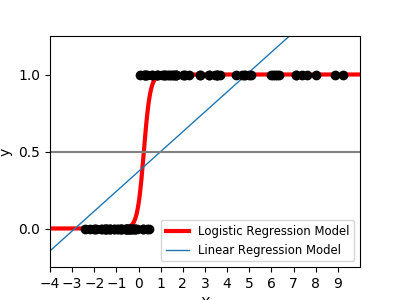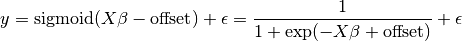>>> logistic = linear_model.LogisticRegression(C=1e5)
>>> logistic.fit(iris_X_train, iris_y_train)
LogisticRegression(C=100000.0, class_weight=None, dual=False,
fit_intercept=True, intercept_scaling=1, max_iter=100,
multi_class='ovr', n_jobs=1, penalty='l2', random_state=None,
solver='liblinear', tol=0.0001, verbose=0, warm_start=False)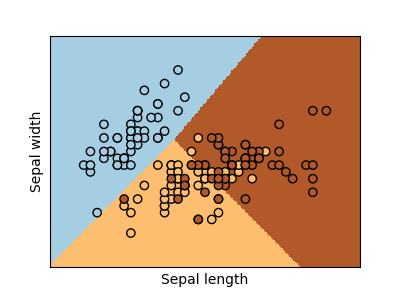LogisticRegression 对象中的 C 参数控制着正则化数量：C 值越大，正则化数量越小。penalty="l2" 提供收缩(比如，无稀疏系数)，同时 penalty=”l1” 提供稀疏化

py from sklearn import datasets, neighbors, linear_model digits = datasets.load_digits() X_digits = digits.data y_digits = digits.target

>[解决方法](https://scikit-learn.org/stable/_downloads/plot_digits_classification_exercise.py)

## 支持向量机(SVMs)

### 线性 SVMs

[支持向量机](5#14-支持向量机) 属于判别模型家族：它们尝试通过找到样例的一个组合来构建一个两类之间最大化的平面。通过 C 参数进行正则化设置：C 的值小意味着边缘是通过分割线周围的所有观测样例进行计算得到的(更正则化)；C 的值大意味着边缘是通过邻近分割线的观测样例计算得到的(更少正则化)。

>例子:
>*   [Plot different SVM classifiers in the iris dataset](https://scikit-learn.org/stable/auto_examples/svm/plot_iris.html#sphx-glr-auto-examples-svm-plot-iris-py)

SVMs 可以用于回归: *SVR* (支持向量回归)–，或者分类 *SVC* (支持向量分类)。

py
>>> from sklearn import svm
>>> svc = svm.SVC(kernel='linear')
>>> svc.fit(iris_X_train, iris_y_train)
SVC(C=1.0, cache_size=200, class_weight=None, coef0=0.0,
decision_function_shape='ovr', degree=3, gamma='auto', kernel='linear',
max_iter=-1, probability=False, random_state=None, shrinking=True,
tol=0.001, verbose=False)



### 使用核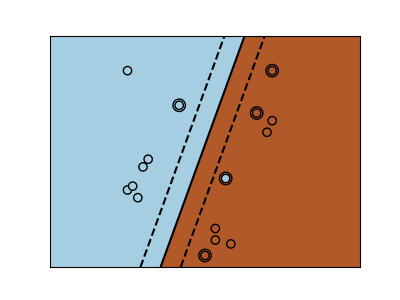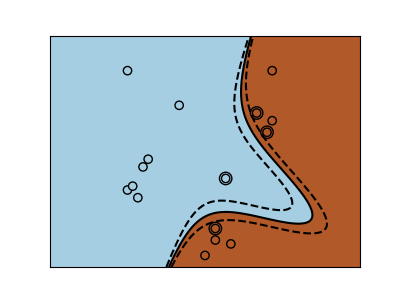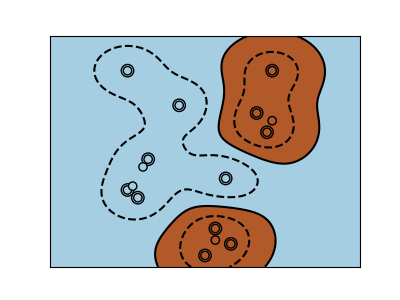>>> svc = svm.SVC(kernel='linear') >>> svc = svm.SVC(kernel='poly',degree=3) >>> svc = svm.SVC(kernel='rbf')